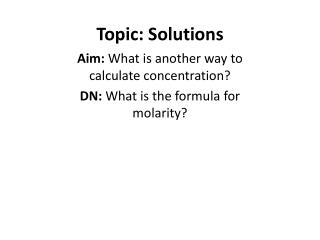Download PresentationTopic: Solutions

# Topic: Solutions

Download Presentation## Topic: Solutions

- - - - - - - - - - - - - - - - - - - - - - - - - - - E N D - - - - - - - - - - - - - - - - - - - - - - - - - - -
##### Presentation Transcript

1. Topic: Solutions Aim: What is another way to calculate concentration? DN: What is the formula for molarity?

2. MOLALITY • Another way of expressing solution concentration • Molality (m) = moles of solute/kg of solvent • http://www.youtube.com/watch?v=96oNrVnTk50 (watch to 1:45) • Demo w/1000mL grad cylinders and stoppers

3. Why/when would we use this? • Youtube video http://www.youtube.com/watch?v=LPj73GjBDkg (what’s the point?) • Molality doesn’t change w/temp of solution. • Solution volume can expand/contract when you heat/cool it • BUT – MASS of solvent won’t change when heated/cooled • SO – the molaRity can change slightly when temp changes, but molaLity won’t. • (explain using example)

4. Ex of molality calculations: a) Calculate the molality of a solution containing 10g NaCl in 500g H2O b) Calculate the molality of a solution containing 50g H2O in 800g ethanol

5. c) Calculate the molality when 75g of MgCl2 is dissolved in 500g of solvent. d) 100g of sucrose (C12H22O11, mol. wt. = 342.3 g/mol) is dissolved in 1.50L of water. What is the molality?

6. e) Determine the molality of 3000g of solvent containing 37.3g of potassium chloride KCl f) How many grams of water must be used to dissolve 100 grams of sucrose C12H22O11 to prepare a .2 molal solution?

7. CHALLENGE: How would you prepare 5000 grams of a .5 molalNaOH solution?

8. How would you prepare 3000g of a .3m NaCl solution? How would you prepare 1000g of a .75m LiCl solution?

9. Colligative Properties • Colligative properties are properties that change when the concentration of the solution changes. • Color, taste, density, BOILING POINT, FREEZING POINT

10. Colligative Properties • When a solute dissolves in a solvent, the BOILING POINT of the resulting solution is ELEVATED from the original boiling point of the solvent by itself. • The increase in the boiling temperature depends on the solute itself and how much of it is added (how concentrated the solution is). • When a solute dissolves in a solvent, the FREEZING POINT of the resulting solution is DEPRESSED from the original boiling point of the solvent by itself. • The decrease in the freezing temperature depends on the solute itself and how much of it is added (how concentrated the solution is).

11. Why does the type of solute used matter when trying to determine how much the boiling point and freezing point will change? • IONIC COMPOUNDS DISSOCIATE (break apart) when they dissolve in water (due to molecule-ion attractions). • The more particles that an ionic compounds splits into, the greater the effect on the boiling point and freezing point. This is called the van’t Hoff factor.

12. van’t Hoff factor • NaCl splits into Na+ and Cl-, or 2 particles. The van’t Hoff factor (i) is 2. • MgCl2splits into Mg2+ and two Cl-, or 3 particles. The van’t Hoff factor (i) is 3. • NaNO3splits into Na+ and NO3-, or 2 particles. The van’t Hoff factor (i) is 2. • C6H12O6 does NOT dissociate because it is MOLECULAR (covalent). The molecules stay together as intact units when the glucose dissolves. The van’t Hoff factor (i) is 1. *This is an oversimplified(!) way of determining the van’t Hoff factor, but it’ll do for our purposes. You’re welcome(?).

13. How to calculate the change in boiling point/freezing point of a solution: Boiling point elevation: ΔTb = iKbm ΔTb = change in the boiling point of the solvent i= van’t Hoff factor Kb = the molal boiling point constant for the solvent (this will be given to you) m = molality of the solution

14. Freezing point depression: ΔTf = iKfm ΔTf= change in the freezing point of the solution i = the van’t Hoff factor Kf = the molal freezing point constant for the solvent (this will be given to you) m = molality of the solution

15. Example calculations of boiling point elevation and freezing point depression: * the Kb of water is .512oC/molal * the Kf of water is -1.86oC/molal

16. Calculate the boiling point elevation of a .3m solution of NaCl(aq). Calculate the freezing point depression of a .3m solution of NaCl(aq).

17. Calculate the boiling point elevation of a .5m solution of KNO3(aq). Calculate the freezing point depression of a .5m solution of C6H12O6(aq).

18. Calculate the boiling point elevation of a solution containing 25g CaCl2 in 500g water.

19. Calculate the freezing point depression of a solution containing 50g C6H12O6 in 500g water.